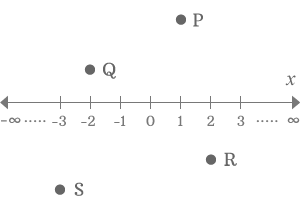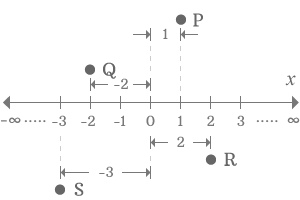x-coordinate

The distance of a point from origin of x-axis in the point of view of same $x$-axis is called $x$-coordinate.

The x-coordinate represents the position of the point on a plane in the point of view of horizontal x-axis. It is measured from the origin of x-axis and the measurement of distance is in the direction parallel to the x-axis. The distance is called x-coordinate in the Cartesian coordinate system.

Example$P,Q,R$ and $S$ are four points on a plane.

1. Point $P$ is above the x-axis and is in positive region.
2. Point $Q$ is also above the x-axis but is in negative region.
3. Point $R$ is below the x-axis and is in positive region.
4. Point $S$ is also below the x-axis but is in negative region.

The four points are at four different locations on the plane. The position of each point can be identified by measuring the distance from origin of x-axis but the measurement of horizontal distance of every point should be in the direction parallel to the x-axis.1. Consider point $P$. It is 1 unit distance from the origin in horizontal direction. Therefore, the x-coordinate of the point $P$ is 1.
2. The point $Q$ is -2 units from the origin of x-axis. So, the x-coordinate of point $Q$ is -2.
3. The point $R$ is 2 units from the origin of x-axis. Therefore, the x-coordinate of point $R$ is 2.
4. The point $S$ is -3 units from the origin of x-axis. Therefore, the x-coordinate of point $S$ is -3.

Latest Math Topics
Latest Math Problems
Email subscription
Math Doubts is a best place to learn mathematics and from basics to advanced scientific level for students, teachers and researchers. Know more